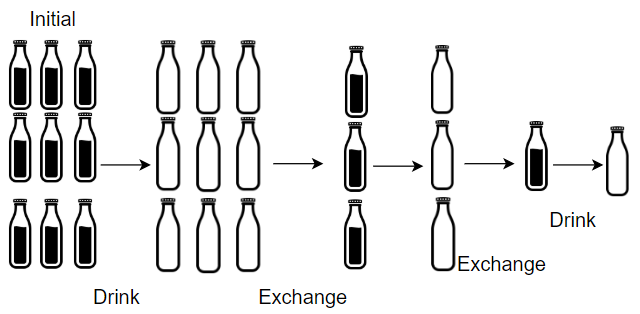1518. Water Bottles

#### QUESTION:

There are numBottles water bottles that are initially full of water. You can exchange numExchange empty water bottles from the market with one full water bottle.

The operation of drinking a full water bottle turns it into an empty bottle.

Given the two integers numBottles and numExchange, return the maximum number of water bottles you can drink.

Example 1:``````Input: numBottles = 9, numExchange = 3
Output: 13
Explanation: You can exchange 3 empty bottles to get 1 full water bottle.
Number of water bottles you can drink: 9 + 3 + 1 = 13.
``````

Example 2:``````Input: numBottles = 15, numExchange = 4
Output: 19
Explanation: You can exchange 4 empty bottles to get 1 full water bottle.
Number of water bottles you can drink: 15 + 3 + 1 = 19.
``````

Constraints:

``````1 <= numBottles <= 100
2 <= numExchange <= 100
``````

#### EXPLANATION:

easy的题目, 其实就和十进制, 二进制的切换相同.

#### SOLUTION:

``````class Solution {
fun numWaterBottles(numBottles: Int, numExchange: Int): Int {
var result = 0
var bottles = numBottles
var carry = 0
while (bottles + carry >= numExchange) {
result += bottles
var tmp = bottles
bottles = (tmp + carry) / numExchange
carry = (tmp + carry) % numExchange
}
return result + bottles
}
}
``````